# Linear Momentum of system of Particles And Conservation of Momentum

## (9) Linear momentum and principle of conservation of linear momentum

• Product of mass and velocity of any particle is defined as the linear momentum of the particle. It is a vector quantity and its direction is same as the direction of velocity of the particle.
• Linear momentum is represented by p. If m is the mass of the particle moving with velocity v then linear momentum of the particle would be
p=mv
like v , p also depends on the frame of reference of the observer.
• If in a many particle system m1 , m2 , m3 , . . . . . . . . . . . . . , mn are the masses and v1 , v2 , v3, . . . . . . . . . . . . ., vn are the velocities of the respective particles then total linear momentum of the system would be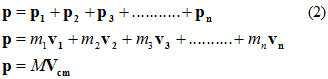where M is the total mass of the system and Vcm is the Velocity of Center of mass of the system
• Hence from equation 2 we came to know that total linear momentum of a many particle system is equal to the product of the total mass of the system and velocity of centre of mass of the system.

• Differentiating equation 2 w.r.t. t we get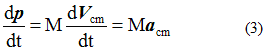but , Macm=Fext which is the external force acting on the system. Therefore,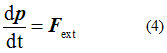like this the rate of change of momentum of a many particle system comes out to be equal to the resultant external force acting on the particle.
• If external force acting on the system is zero then,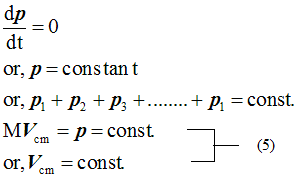• Hence we conclude that when resultant external force acting on any particle is zero then total linear momentum of the system remains constant. This is known as law of conservation of linear momentum.
• Above equation 5 is equivalent to following scalar quantities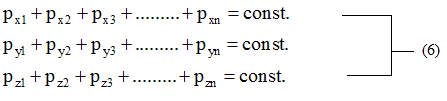Equation 6 shows the total linear momentum of the system in terms of x , y and z co-ordinates and also shows that they remain constant or conserved in absence of any externally applied force.
• The law of conservation of linear momentum is the fundamental and exact law of nature. No violation of it has ever been found. This law has been established on the basis of Newton's law but this law holds true in the situations where Newtonian mechanics fails.